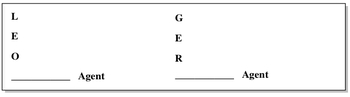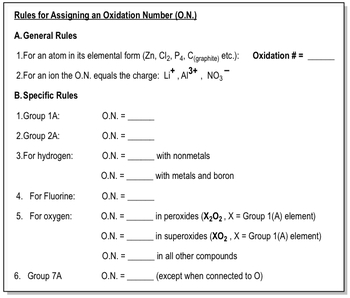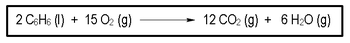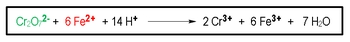Start typing, then use the up and down arrows to select an option from the list.## General Chemistry

Learn the toughest concepts covered in Chemistry with step-by-step video tutorials and practice problems by world-class tutors

20. Electrochemistry

Oxidation and reduction deals with the transferring of electrons between reactants. The reactant that loses electrons is oxidized, while the reactant that gains electrons is reduced

Redox Reactions
1
concept

## Understanding Oxidation versus Reduction1m
Play a video:
we're gonna say chemists usually use important terminology to describe the movement of electrons. Remember, electrons are the negative subatomic particles we're going to say in Redox reactions, Redox reactions. We have the movement of electrons from one reactant toe. Another reacted. Now we're gonna use ah, funny type of sentence to help us remember what's going on. And the sentences. Leo the lion goes ger might sound corny, but trust me, it helps a lot with understanding, oxidation and reduction. So we said Leo, the lion goes girl. So Leo, lose electrons. Oxidation goes, girl gain electrons reduction. Now remember, electrons are negatively charged. So if you're losing negatively charged subatomic particles, you're becoming more positive. So lose electrons means you're becoming mawr positive. Okay, if you're gaining negative electrons, you're gaining negative things. So you are gonna become more negative. Okay? And then here's the word thing. If you've been oxidized, if you want to go oxidation, you're called the reducing agent. Go, Go. Okay, so you're the reducing agent. If you've been reduced, then you're the oxidizing agentWhen in doubt about oxidation or reduction just remember the phrase:"LEO the lion goes GER."

2
concept

## Rules for Oxidation Numbers4m
Play a video:
Now that we know the distinction between oxidation and reduction, it's important to set down some ground rules toe help us figure out what's the particular oxidation state of a particular element. So I break rules for assigning oxidation numbers, which we're gonna abbreviate O n so oxidation numbers o n I break it down into two sets of rules. We have our two general rules, and then we have arm or specific rules. Now, the two general rules, we're gonna say, for an Adam in its elemental form, what is elemental for me. While elemental form means two things, it means that the metal is by itself, such as sodium, sodium metal is by itself or the element is connected to itself. Oxygen here is connected to another oxygen sulfur here is connected to a bunch of sulfur is just like itself. So if you're by yourself or you're connected to copies of yourself and you have no charge, that is your elemental form. So let's write that down. Elemental form means you are by yourself or connected two copies of yourself and you have no charge. So if you fit these types of categories, then you're oxidation numbers equal to zero. Not the second general rule is for an eye on whatever the ions charges. That's it's oxidation number, so sodium here is by itself, but it has a plus one charge, so it's oxidation. Number is plus one. Calcium is by itself, but it has a plus to charge. So it's oxidation numbers plus two. Now No. Three minus. That's our nitrate ion, our Polly atomic ion. The oxidation number for the entire compound is negative one. Now, if we want to find the oxidation state of each of the individual elements, that's when we move on to our specific rules. Now are specific rules. We're gonna say Group one A plus one group to eight plus two, based on the charge distributions that we learned earlier. But we're going to save the hydrogen. Hydrogen is in Group one A. But hydrogen is not always plus one. We're gonna say it's plus one when it's connected to non metals. So if it's connected to si, ahl or perk loit or in water and these three examples water is connected. Toa Onley nonmetal, so it's plus one. But if hydrogen is connected to ah, metal or blond, it's minus one. So if we had any H or B H three than in those cases, hydrogen would be negative one. We're gonna say Florida is always minus one, no matter what. Oxygen, on the other hand, can be a pain because oxygen has various oxidation states, and it's all based on what kind of compound it's in. So we're gonna say for oxygen. When it's it's ah peroxide, it's gonna be negative one. Now, what exactly is a peroxide? Ah, peroxide has the formula X two zero two X equals a group one a element. So hydrogen, lithium, potassium, all those air group won eight medals. So a good example. Here you could have hydrogen peroxide or sodium peroxide. Both are peroxide because both have the formula X two o two. In this case, oxygen would be minus one. Now oxygen will be negative. A half when it's a super oxide. Ah, super oxide has the formula X o two X again is a group on a element. So, for example, you could have sodium super oxide or lithium super oxide. Now we're gonna basically say if oxygen is not a peroxide or super oxide weaken. Say that it's minus two. And this is the normal state of oxygen because we sell them. See peroxide or super oxides. But if we do not see one of those to remember oxygen, we minus two now groups seven eight elements are halogen. They're gonna be minus one. Except when they're connected to oxygen when they're connected. Toa oxygen. We're gonna have to use some mathematical knowledge in order to do it. Simple algebra. We'll get to see how this works when we do practice questions dealing with this concept. So remember, we have our two general rules, and then we have arm or specific rules.We use these oxidation number rules whenever we are asked to determine the oxidation number of an entire compound or the individual elements within a compound.

3
example

## What is the oxidation number of each underlined element2m
Play a video:
Now, Now that we've seen this, let's take a look at the example. It says, What is the oxidation number off each underlying element For the first one, we have I to so remember which rules should we look at? Well, here we have nonmetal. We have an element connected to a copy of itself, and it has no charge. Remember, when we see this, the oxidation numbers automatically equal to zero. Come for the next one. We have C s to go to remember, this has the formula X to go to. And cesium is a group one element. So this would be a peroxide, and therefore the oxidation number of oxygen would be minus one. Okay. And then finally, the last one here. I don't specify which one we wanna find. I'm gonna tell it to you now. It's carbon. I want us to find carbon. Now, I'm gonna take myself out of the shot guy. So we have more room to work this out together. Okay, so we want to find the oxidation number off carbon. The thing is, we never came up with a rule for carbon. It's nowhere in the specific rules. So because of that we don't know what it is. And therefore it's X hydrogen. We do have a rule for it. When it's connected to non metals, it's plus one. This compound doesn't take the form of ah peroxide. It doesn't look like this. It doesn't take on the form of a super oxide. It doesn't look like this. Therefore, oxygen must be minus 2/4 oxidation state. Now what we're gonna do here is we're gonna use simple algebra to help us software the missing variable. So we have one hydrogen, that is plus one. There's one carbon, which is X plus. There are three oxygen's. Each one is minus two. This equation will equal the charge of the compound. Now the charge of the compound is minus one. Now, we're just gonna solve for the missing variable. So we're gonna have one plus x three times. Negative two gives me negative six equals minus one. The positive one in the negative six will combine to give me negative five. We still want to find X, so we add five to both sides. So the oxidation state of carbon would be plus four

Instead of being asked who is oxidized or reduced, sometimes you may be asked to determine the reducing and oxidizing agents

4
example

## In the following reaction identify the oxidizing agent and the reducing agent5m
Play a video:
Welcome back, guys. In this new video we're gonna practice on How do we identify the oxidation numbers of certain compounds based on the rules that we learned about redox reactions. So let's take a look. We say this example in the following reaction identified the oxidizing agent and the reducing agent. So let's take a look here. We're gonna first try to identify the easiest portions of this we're gonna say, Here we have 02 We have an element connected to a copy of itself, and it has no charge, so the oxidation number of oxygen here will be zero. Next, we're gonna look at the hydrogen. Hydrogen here is connected to a non metal, which is carbon. Therefore, it's oxidation. State is plus one. Now, we don't have a rule for carbon here, So we're just gonna say that carbon here is X and we're gonna use a mathematical type of strategy in order to solve for that missing variable. Remember, when we're looking for the oxidation state of a particular element, ignore the coefficient coefficient is not important. So we're going to say we have six carbons, so six x plus six hydrogen is each one is plus one equals the charge of the compound. The charge of the compound is zero. I don't say anything about it being positive or negative in this space here. So we just say zero now six X plus six equals zero. We're gonna say Subtract six from both sides. So six X equals negative six. Divide by six X equals negative one. So the oxidation state of carbon, you ought to be minus one. Next, we're gonna go to the other side. Here we know that hydrogen is connected to oxygen, which is a non metal. So therefore it's plus one. This structure is not ah peroxide or a super oxide. Remember, we still need to know that so oxygen, who will be minus two Co two? This is not a super oxide because remember, super oxide does have the form of X 202 But acts needs to be a group. When a element carbon is not a group on a element. It's a group for a element. Therefore, oxygen here is minus two. Now we have to solve for carbon again. So it's X and remember it's X because we don't have any type of role for it. We don't have a general rule or specific role for it. Okay, so this right here, ignore the coefficient. So now we just saw for we're gonna say X because there's only one carbon plus two oxygen's. Each one is minus two equals that charge the compound, which is zero x minus four, equals zero at four to both sides. X equals plus four. Now we have to identify the oxidizing agent and the reducing agent. Remember to do that, we have to remember Leo the lion goes ger. Okay, so we're looking to see who became more positive and who became more negative where we go from its reacting form to its product form. So if we take a look at carbon, we're gonna say Carbon starts out as negative one when it's reacted. Then when it becomes a product, it's oxidation state goes to plus four. So we're gonna say carbon became or positive because it goes from negative 12 plus four. Since it became more positive, it was oxidized. If you were oxidized, then you're the reducing agent. So that's the type of mindset you have to have when doing these types of questions does the oxidation state become more positive or more negative? As you go from being a reactant to becoming a product, who else changes where we're going to say that Oxygen 02 goes from being zero to being negative too? It doesn't matter which oxygen we look look at for products because they're both minus two for their oxidation states. So we're gonna say oxygen goes from zero to negative two. It became more negative. Therefore it was reduced. And if it was reduced, its the oxidizing agent Mhm. So that's the pattern you have to follow in order to solve this type of question. Remember, it's all based off of Leo. The lion goes ger Leo, lose electrons, oxidation, ger, gain electrons reduction. Go back to the previous concept video and look at how we manage that chart. And just use it to solve this question. Okay, guys, the next to set of questions on the same page, our practice. So I want you guys to attempt on doing it on your own. Then come back and look at the video where explained how we solve both of these practice questions. Good luckTry your skills at determing the oxidation number of elements within molecules or polyatomic ions.

5
Problem

What is the oxidation number of each underlined element?

a) P4                                  b) BO33–                                c) AsO42–                           d) HSO4

Just remember if you've been oxidized then you're the reducing agent and if you've been reduced then you're the oxidizing agent.

6
Problem

In the following reaction identify the oxidizing agent and the reducing agent:

Cr2O72- + 6 Fe2+ + 14 H→ 2 Cr3+ + 6 Fe3+ + 7 H2OBasic Redox Concepts
7
concept

## Basic Redox Concepts 13m
Play a video:
so recall that oxidation reduction reactions or redox reactions involves the transferring of electrons from one reactant toe another. Now here in our equation, we have lithium solid reacting with chlorine gas, and in this process we produce lithium ion as well as to chloride ions. Now, remember, when it comes to Redox reactions, we have oxidation and reduction to help us remember key concepts in terms of those two words we use Leo, the lion goes ger. Remember, Leo means that we're losing electrons and therefore represent oxidation. When you're losing negatively charged electrons, the species or element in this case is becoming more positive. That's because it's losing something negative now. What exactly about that element is becoming more positive? Well, it's oxidation. Number is becoming more positive. As a result. We're gonna say here that if you are being oxidized, then you represent the reducing agent or the reductive. In this case, we have lithium solid in its natural state, so it's oxidation. Number is equal to zero now, as an ion, it's oxidation. Number is tied to its charge, so here it be plus one. So for lithium, it goes from being zero to plus one so it's oxidation number has increased. Therefore it has been oxidized. Therefore, it is the reducing agent or the reductive on the other side. When we gain, electrons were gaining negative electrons. That means the species or the element in this case is becoming more negative. As a result of this, the oxidation number decreases here. If you are being reduced, then you represent the oxidizing agent or the Occident. In this case, C L two gas it's in. It's natural or elemental state, so it's oxidation numbers equal to zero. Now, as an ion, it's oxidation numbers tied to its charge. Each chloride ion is minus one, so each chloride ion is minus one in terms of oxidation. Together they would be minus two. We would say here that we said lithium was being oxidized, so it's losing electrons. It loses. In this case, technically would lose two electrons and chlorine itself would pick up those two electrons because each chlorine needs to gain an electron so each chlorine becomes a chloride ion. As you go deeper, deeper into redox, reactions will talk about concepts such a self potential, the use of electrochemical cells as off as well as other concepts related to charge or voltage. Now that you've gotten down the basics of Redox reactions, click on the next video and see the different types of variables tied to the transferring of electrons between reactions.
8
concept

## Basic Redox Concepts 27m
Play a video: